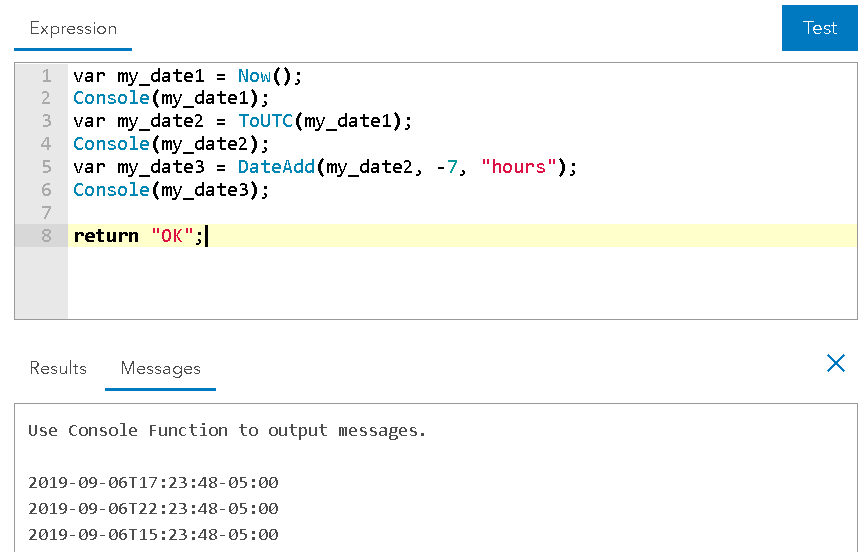# Arcade Expression Converting Text to Date

1578
6
09-05-2019 08:09 AMOccasional Contributor II

I am seeing if anyone has a solution for converting text to date that is in the following format 201909050425 within Arcade Expressions?

Tags (1)
6 RepliesbyEsri Regular Contributor

Hi Eric Shreve‌,

I think you could probably modify the Arcade expression found in this thread to convert the string to a date:

Hope this helps,

PeterbyEsri Esteemed Contributor

Hi Eric Shreve ,

What is the format of the data? Is 201909050425 in YYYYMMDDHHmm format or is it perhaps YYYYDDMMHHmm?

In case it is first month and then day, it would be something like this (assuming the you are using military hours):

``````// Date ( value , month , day , hour , minute , second , millisecond )
var txt = "201909050425";

var YY = Number(Mid(txt, 0 , 4));
var MM = Number(Mid(txt, 4 , 2)) - 1;
var DD = Number(Mid(txt, 6 , 2));
var HH = Number(Mid(txt, 8 , 2));
var mi = Number(Right(txt, 2));

// Console(YY);
// Console(MM);
// Console(DD);
// Console(HH);
// Console(mi);

return Date(YY, MM, DD, HH, mi);‍‍‍‍‍‍‍‍‍‍‍‍‍‍‍‍``````Occasional Contributor II

It is formatted as YYYYMMDD. I switched the 'var txt' to 'VALID' which is the the field in the feature service and I am not able to get it working. Do you know what I am missing in the configuration?

``````// Date ( value , month , day , hour , minute , second , millisecond )
var txt = "VALID";

var YY = Number(Mid(txt, 0 , 4));
var MM = Number(Mid(txt, 4 , 2)) - 1;
var DD = Number(Mid(txt, 6 , 2));
var HH = Number(Mid(txt, 8 , 2));
var mi = Number(Right(txt, 2));

// Console(YY);
// Console(MM);
// Console(DD);
// Console(HH);
// Console(mi);

return Date(YY, MM, DD, HH, mi);‍‍‍‍‍‍‍‍‍‍‍‍‍‍‍‍``````

Thanks,

Eric ShrevebyEsri Esteemed Contributor

I don't think it is formatted as "YYYYMMDD" since you have more numbers, or are you only interested in the date and not the time?

In case your format is "YYYMMDDHHmm" you would use this:

``````var txt = \$feature.VALID; // in case your field is called "VALID"

var YY = Number(Mid(txt, 0 , 4));
var MM = Number(Mid(txt, 4 , 2)) - 1;
var DD = Number(Mid(txt, 6 , 2));
var HH = Number(Mid(txt, 8 , 2));
var mi = Number(Right(txt, 2));

return Date(YY, MM, DD, HH, mi);‍‍‍‍‍‍‍‍‍``````

In case you are only interested in the date not the time, you would use this:

``````var txt = \$feature.VALID; // in case your field is called "VALID"

var YY = Number(Mid(txt, 0 , 4));
var MM = Number(Mid(txt, 4 , 2)) - 1;
var DD = Number(Mid(txt, 6 , 2));

return Date(YY, MM, DD);‍‍‍‍‍‍‍‍‍‍‍‍‍‍‍‍``````Occasional Contributor II

How would I offset the time to local time?

I tried adding this line of code but it looks like it is not taking:

var pacificTime = DateAdd(ToUTC(Date), -7, "hours");byEsri Esteemed Contributor

Your expression will not work, since you are using "Date" which is not a value, but an existing function. Have a look below: I create a date with the function Now, Then I convert to UTC with ToUTC and I apply the DateAdd function. All intermediate results are written to the console (messages part) and you can see the different date-time results.